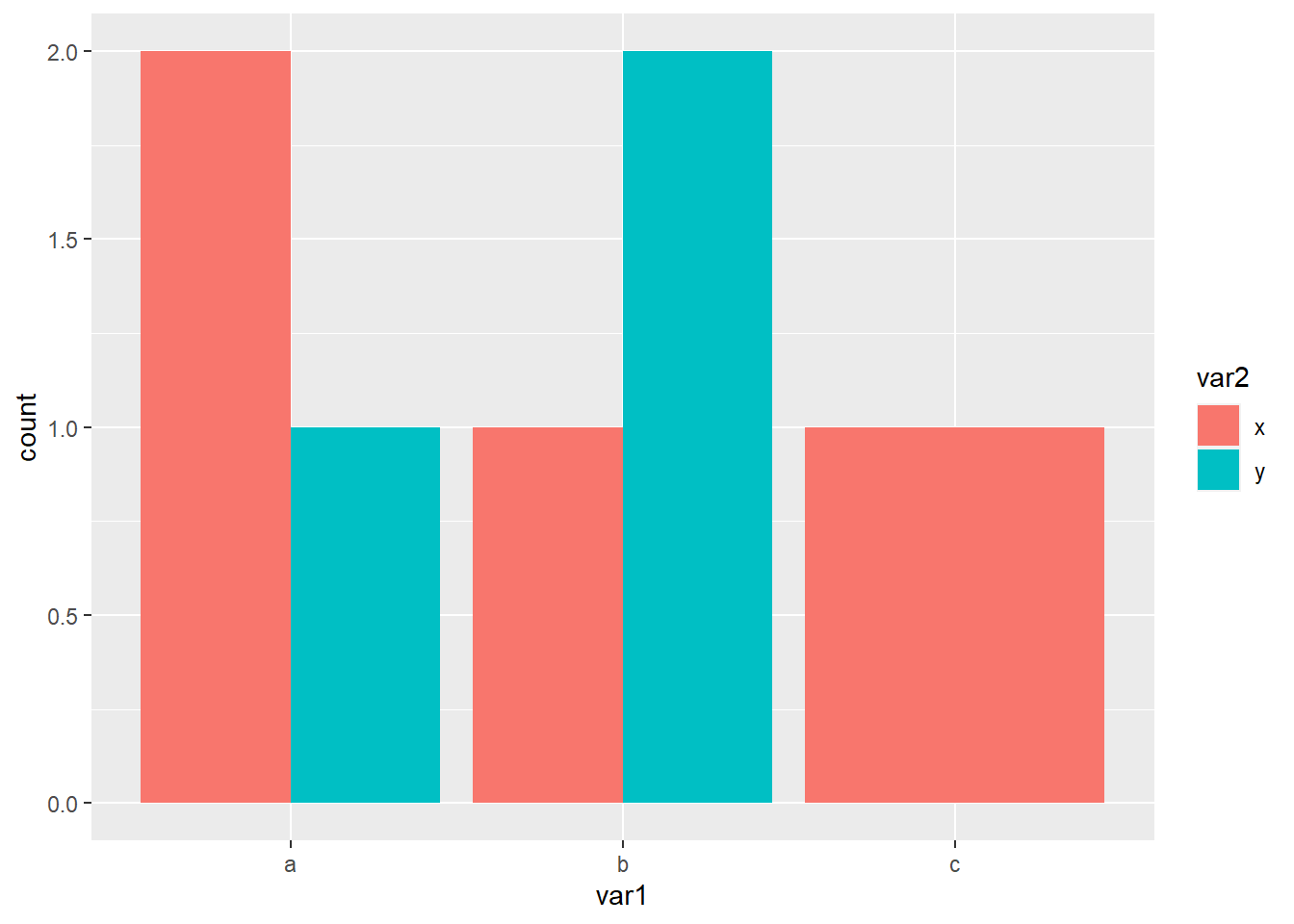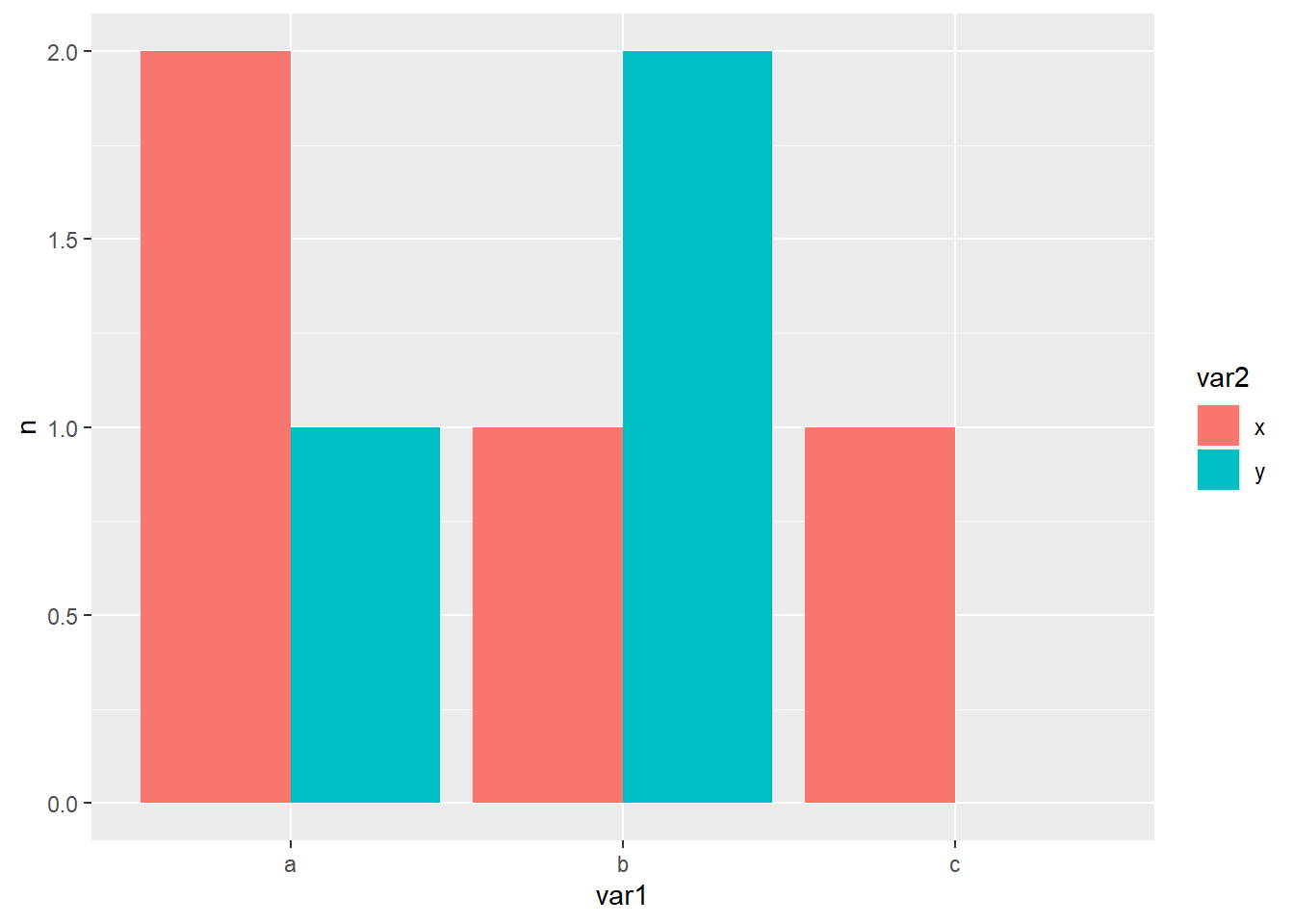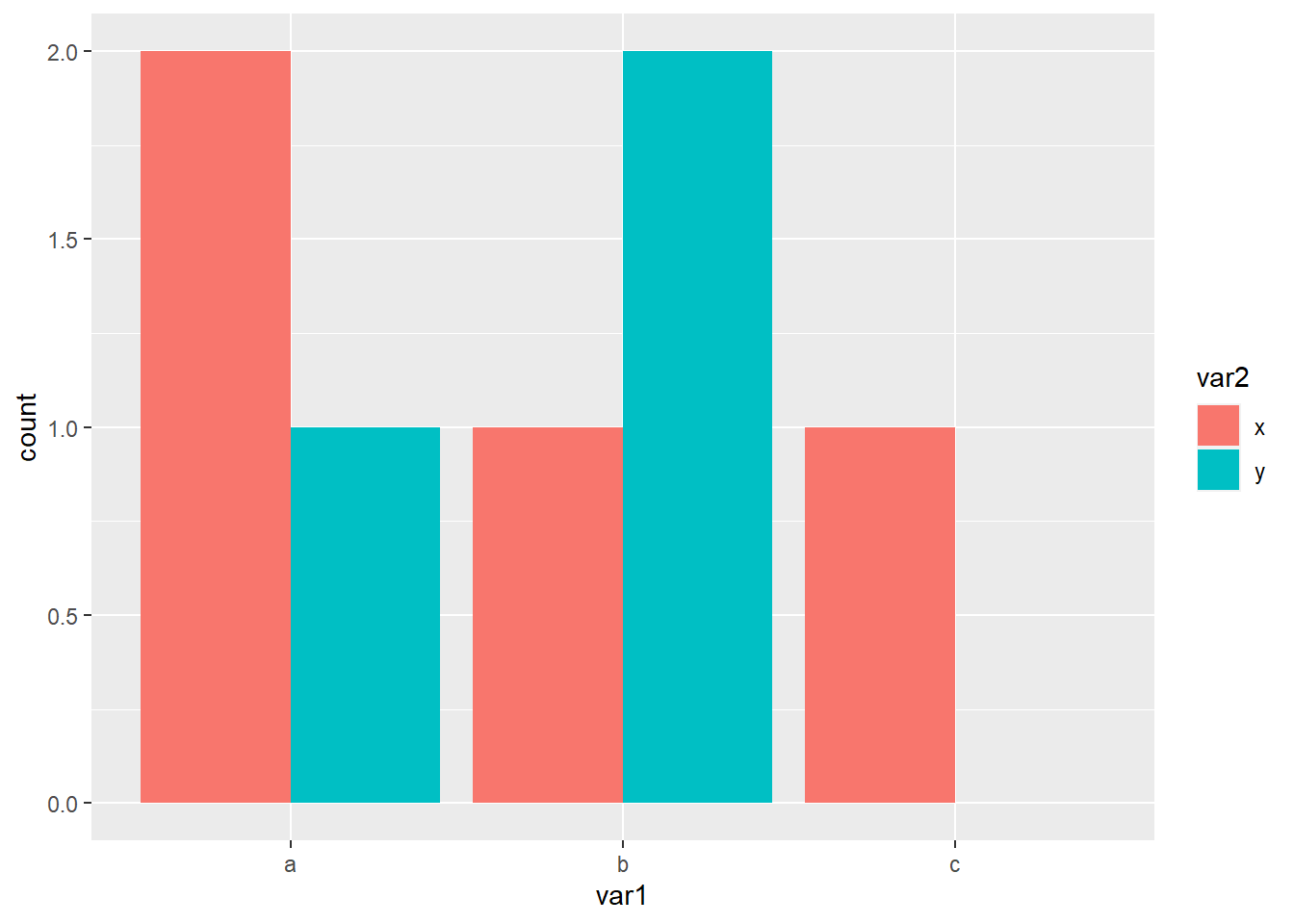### Dealing with missing data in ggplot2 barplots

#### September 20, 2017    ggplot2geom_bar()

I’m wrting this down because it’s a problem I’ve tried to deal with twice in the last couple of weeks and I had to look the solution up both times. The second time, rather than just fix it I dug a little deeper to find out why something I thought would work didn’t.

Any way, the problem:

library(ggplot2)
fake_data <- data.frame(var1 = c("a", "a", "a", "b", "b", "b", "c"),
var2 = c("x", "x", "y", "x", "y", "y", "x"))
fake_data 
  var1 var2
1    a    x
2    a    x
3    a    y
4    b    x
5    b    y
6    b    y
7    c    x
ggplot(fake_data, aes(x = var1, fill = var2)) +
geom_bar(position = "dodge")Now that all works fine but I don’t want the bar for c to be full width, just half width as the others are, so the missing data (or count of zero is clearer).

This same problem has been dealt with in two stackoverflow posts1 2 and Hadley Wickham (the package author) commented on the design of the position_dodge() in this issue. The basic idea is to stop ggplot from dropping the missing bar (or bar with count 0).

ggplot(fake_data, aes(x = var1, fill = var2)) +
geom_bar(position = "dodge") +
scale_x_discrete(drop = FALSE) +
scale_fill_discrete(drop = FALSE)As you can see this makes no difference. The reason is because all the solutions count the data before plotting and use stat = "identity" and I wondered why that was necessary, why wouldn’t it work without it. To get the answer I had a look at the plot in more detail.

p = ggplot(fake_data, aes(x = var1, fill = var2)) +
geom_bar(position = "dodge")
ggplot_build(p)\$data
[]
fill y count prop     x flipped_aes PANEL group ymin ymax xmin xmax colour
1 #F8766D 2     2    1 0.775       FALSE     1     1    0    2 0.55 1.00     NA
2 #00BFC4 1     1    1 1.225       FALSE     1     2    0    1 1.00 1.45     NA
3 #F8766D 1     1    1 1.775       FALSE     1     3    0    1 1.55 2.00     NA
4 #00BFC4 2     2    1 2.225       FALSE     1     4    0    2 2.00 2.45     NA
5 #F8766D 1     1    1 3.000       FALSE     1     5    0    1 2.55 3.45     NA
size linetype alpha
1  0.5        1    NA
2  0.5        1    NA
3  0.5        1    NA
4  0.5        1    NA
5  0.5        1    NA

The data only has 5 rows and so there isn’t any data to stop ggplot from dropping. It is necessary to count the data first.

# starting again
library(tidyverse)
fake_data <- data.frame(var1 = c("a", "a", "a", "b", "b", "b", "c"),
var2 = c("x", "x", "y", "x", "y", "y", "x"))
# count the data
count_fake = count(fake_data, var1, var2)
# fill in the missing combination
count_fake = complete(count_fake, var1, var2)
# plot the data
ggplot(count_fake, aes(x = var1, y = n, fill = var2)) +
geom_bar(stat = "identity", position = "dodge")Note: In the development version of ggplot2 there is a much easier solution. First we need to install the development version (presuming you have already installed devtools.)

devtools::install_github("tidyverse/ggplot2")

Then we have access to an additional argument within position_dodge().

library(ggplot2)
fake_data <- data.frame(var1 = c("a", "a", "a", "b", "b", "b", "c"),
var2 = c("x", "x", "y", "x", "y", "y", "x"))
ggplot(fake_data, aes(x = var1, fill = var2)) +
geom_bar(position = position_dodge(preserve = 'single'))